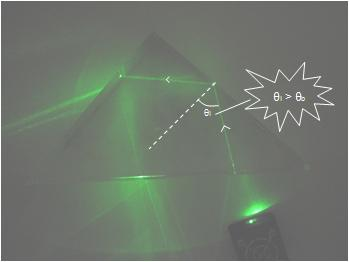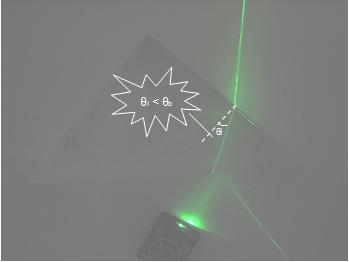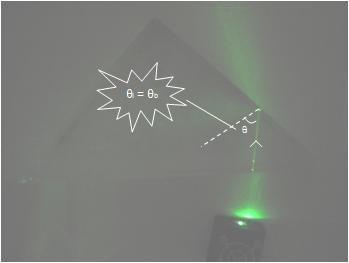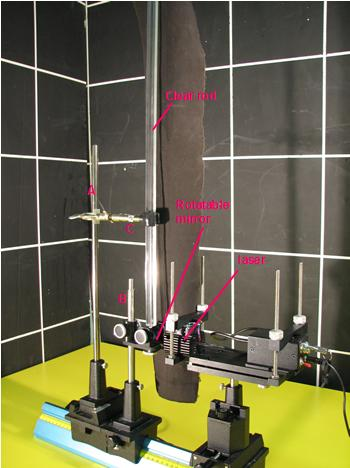### A great way to show Total Internal Reflection and how it works!

WATCH VIDEO:

Teachable Topics:

• Derivation of critical angle from Snell’s law
• Refraction
• Reflection
• Fiber optics
• Snell’s law

Theory:

The phenomenon shown in this demonstration is put to use every day with various applications of fiber optics. Total internal reflection is due to the properties of the materials the light is traveling through and the concept of the critical angle. If light hits a surface at an angle larger than the critical angle, it will be reflected (see Fig.1); if light hits a surface at an angle smaller than the critical angle, it will be refracted (see Fig.2). Finally, if light hits a surface at an incident angle equal to the critical angle, the angle of transmittance will be 90° to the normal (see Fig. 3). The critical angle is unique to each material and depends on its index of refraction and also the index of refraction of the medium the incident light is in.

From Snell’s law, it is found that the critical angle can be calculated as follows:

sinqb = ns / nm

where qb = Critical angle, ns = Substance’s index of refraction, nm = Incident medium’s index of refraction

In this demonstration, we are rotating the mirror through the extent of what is called the “cone-angle”. The cone angle is essentially a 3D representation of where the critical angle occurs. It is the region which the angle of incidence must be smaller than for refraction to occurFigure 1: Internal ReflectionFigure 2: RefractionFigure 3: Critical angle

Apparatus:

• laser (λ = 532.8nm was used in this demonstration) and mount
• small, rotatable mirror attached to a small metal rod on mount (B)
• larger metal rod on mount (A)
• clamp (C)
• long, rectangular, straight, clear rod
• optical bench
• dark fabric (optional)Figure 4: Set-up of internal reflection demonstrator

Procedure:

• Set up apparatus as shown in Figure 1
• Adjust the apparatus until you can get the laser reflecting off the mirror directly up the length of the clear rod. This may take some fine adjustments and time
• Slowly move the orientation of the mirror and watch the laser reflect off the inside surfaces of the rod

SAFETY WARNINGS:

• Ensure that no one is in the path of the reflected laser at any orientation of the mirror.